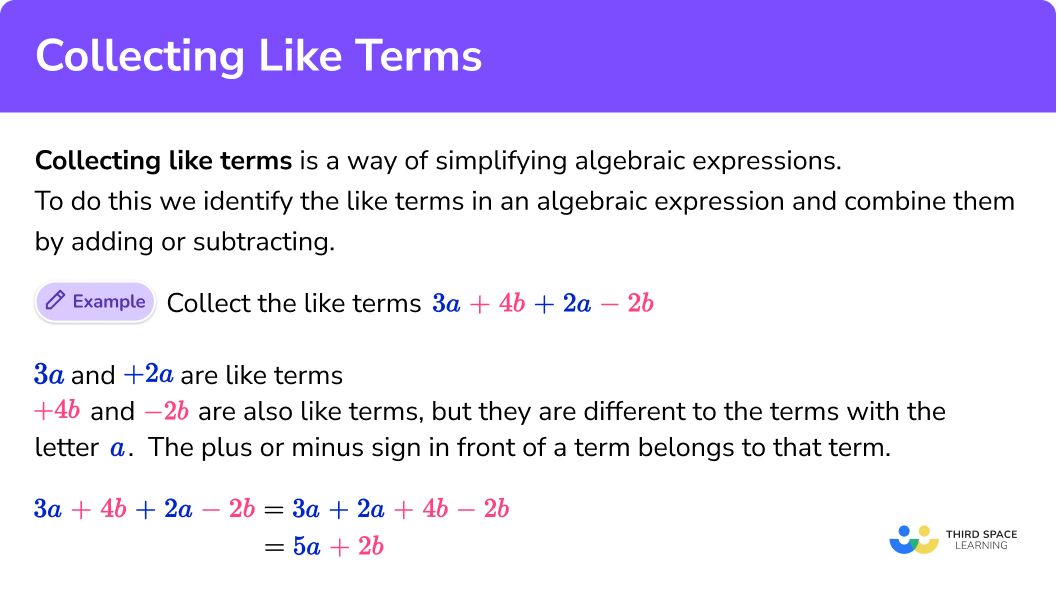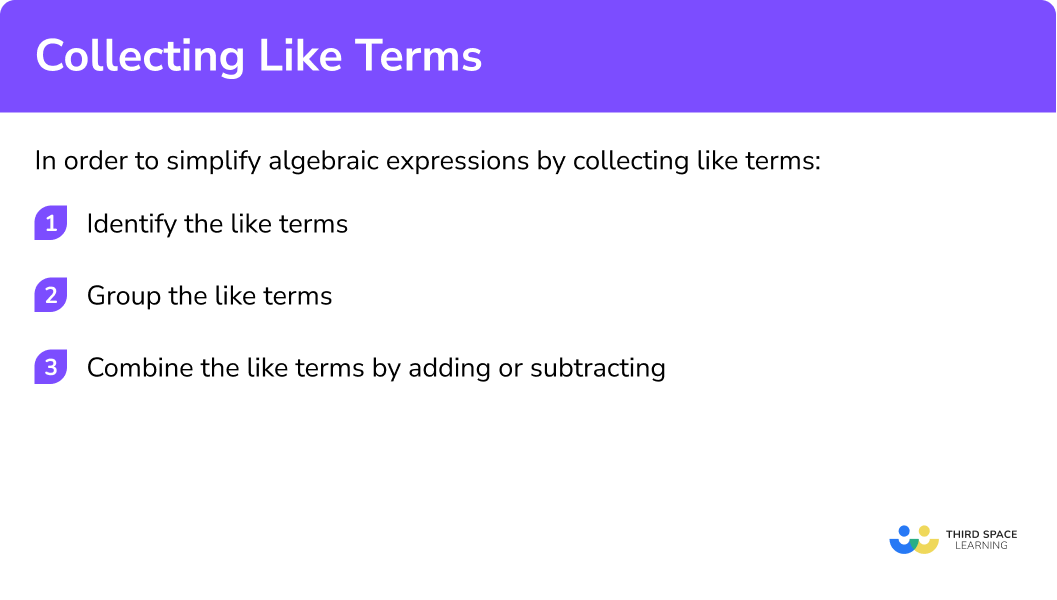# Collecting Like Terms

Here we will learn about simplifying algebraic expressions by collecting like terms

There are also collecting like terms worksheets based on Edexcel, AQA and OCR exam questions, along with further guidance on where to go next if you’re still stuck.

## What do we mean by collecting like terms?

Collecting like terms is a way of simplifying algebraic expressions. It is also known as combining like terms.
To do this we identify the like terms in an algebraic expression and combine them by adding or subtracting.

E.g.
This is an expression with 4 terms:

$3a +4b + 2a -2b$

3a and +2a are like terms (they have the same letter).
+4b and -2b are also like terms, but they are different to the terms with the letter a.
The plus or minus sign in front of a term belongs to that term.

\begin{align*} 3a +4b + 2a – b &= 3a + 2a+ 4b – b\\ &=5a+2b\\ \end{align*}

This expression cannot be simplified any more as the 5a and the +2b are unlike terms.

### What do we mean by collecting like terms?## How to collect like terms

In order to simplify algebraic expressions by collecting like terms:

1. Identify the like terms
2. Group the like terms
3. Combine the like terms by adding or subtracting

### Explain how to collect like terms## Collecting like terms examples

### Example 1: one type of like term

Simplify:

$3x+2x +4x$

1. Identify the like terms

All the terms involve an x. They are all the same type of term.

$3x+2x +4x$

2Group the like terms

The like terms are already grouped together.

$3x+2x +4x$

3Combine the like terms by adding or subtracting

$3x+2x +4x =9x$

$9x$

### Example 2: one type of like term

Simplify:

$6a-3a +4a$

All the terms involve an a. They are all the same type of term.

$6a-3a +4a$

The like terms are already grouped together.

$6a-3a +4a$
$6a-3a +4a=7a$

Because

$6-3+4=7$

$7a$

### Example 3: two types of like terms

Simplify:

$7a+4b+2a+3b$

The terms involving a are like terms (7a and +2a)
The terms involving b are like terms (+4b and +3b)
The plus (or minus) sign belongs to the term after it.

$7a+4b+2a+3b=7a+2a+4b+3b$
\begin{align*} 7a+4b+2a+3b &=7a+2a+4b+3b\\ &=9a+7b\\ \end{align*}

Because

$7+2=9$

And

$4+3=7$

$9a+7b$

### Example 4: two types of like terms

Simplify the linear expression:

$5x+4y-3x+5y$

The terms involving x are like terms (5x and -3x)
The terms involving y are like terms ( +4y and +5y)
The plus or minus sign belongs to the term after it.

$5x+4y-3x+5y=5x-3x+4y+5y$
\begin{align*} 5x+4y-3x+5y &=5x-3x+4y+5y\\ &=2x+9y\\ \end{align*}

Because

$5-3=2$

And

$4+5=9$

$2x+9y$

### Example 5: three types of like terms

Simplify the algebraic terms:

$2c-5d+4+3c+7d+2$

The terms involving c are like terms (2c and +3c)
The terms involving d are like terms (-5d and +7d)
The terms that are only numbers are known as constants (+4 and +2)
The plus or minus sign belongs to the term after it.

$2c-5d+4+3c+7d+2= 2c+3c-5d+7d+4+2$
\begin{align*} 2c-5d+4+3c+7d+2 &=2c+3c-5d+7d+4+2\\ &=5c+2d+6\\ \end{align*}

Because

$2+3=5$

And

$-5+7=2$

And

$4+2=6$

$5c+2d+6$

### Example 6: three types of like terms

Simplify:

$-6x+5y+4+2x-3y-7$

The terms involving x are like terms (-6x and +2x)
The terms involving y are like terms (+5y and -3y)
The terms that are only numbers are known as constants (+4 and -7)
The plus or minus sign belongs to the term after it.

$-6x+5y+4+2x-3y-7= -6x+2x+5y-3y+4-7$
\begin{align*} -6x+5y+4+2x-3y-7 &=-6x+2x+5y-3y+4-7\\ &=-4x+3y-3\\ \end{align*}

Because

$-6+2=-4$

And

$5-3=2$

And

$4-7=-3$

$-4x+2y-3$

### Example 7: variables with exponents

Simplify:

$8x^{2}+5x-4x^{2}+7x$

The terms involving x2 are like terms (8x^{2} and -4x^{2})
The terms involving x are like terms (+5x and +7x)
The plus or minus sign belongs to the term after it.

$8x^{2}+5x-4x^{2}+7x = 8x^{2}-4x^{2}+5x+7x$
\begin{align*} 8x^{2}+5x-4x^{2}+7x &= 8x^{2}-4x^{2}+5x+7x\\ &=4x^{2}+12x\\ \end{align*}

Because

$8-4=4$

And

$5+7=12$

$4x^{2}+12x$

### Example 8: variables with exponents

Simplify:

$6x^{2}y-2x^{2}+4x^{2}y-5x^{2}$

The terms involving x2y are like terms (6x^{2}y and +4x^{2}y)
The terms involving x2 are like terms (-2x^{2} and -5x^{2})
The plus or minus sign belongs to the term after it.

$6x^{2}y-2x^{2}+4x^{2}y-5x^{2}=6x^{2}y+4x^{2}y-2x^{2}-5x^{2}$
\begin{align*} 6x^{2}y-2x^{2}+4x^{2}y-5x^{2}&=6x^{2}y+4x^{2}y-2x^{2}-5x^{2}\\ &=10x^{2}y-7x^{2}\\ \end{align*}

Because

$6+4=10$

And

$-2-5=-7$

$10x^{2}y-7x^{2}$

### Common misconceptions

• No coefficient (number) seen in front of a term

If there is no coefficient (number) seen in front of a term then the coefficient is 1, but we do not write the number 1.

$x+4x=1x+4x=5x$

• When asked to simplify an algebraic expression it is possible for the answer to be zero

It is possible for all the terms to be cancelled out and the answer is zero.

E.g.

$5x+3y-5x+4y=7y$

The terms involving x have cancelled out. We do not write 0x.

• Terms must be exactly the same to be “like terms”

Terms involving y and y2 are unlike terms and cannot be collected together by adding or subtracting.

$3y+4y^2+2y=5y+4y^2$

This has been simplified as far as possible.

• The order of the terms in the final answer is not important

The order of the terms is not critical as long as the plus and minus signs are with the correct term.

So:

$3m+4n=4n+3m$

And:

$-2x+5y=5y-2x$

### Practice collecting like terms questions

1. Simplify:

4x+2x+3x

9x11x8x9xxx4x+2x+3x=9x

Because:

4+2+3=9

2. Simplify:

3a-2a+4a

5a^39a^39a5a3a-2a+4a=5a

Because:

3-2+4=5

3. Simplify:

5c+3d-2c+4d

7c+7d3c+7d7c-d10cd\begin{aligned} 5c+3d-2c+4d &=5c-2c+3d+4d\\ &=3c+7d\\ \end{aligned}

4. Simplify:

7x-2y-4x+5y

3x+3y11x+7y3x+7y6xy\begin{aligned} 7x-2y-4x+5y &=7x-4x-2y+5y\\ &=3x+3y\\ \end{aligned}

5. Simplify:

2m+3n+1+4m+5n+6

5m+5n+116m+8n+721mn5m+7n+7\begin{aligned} 2m+3n+1+4m+5n+6 &=2m+3n+1+4m+5n+6\\ &=6m+8n+7\\ \end{aligned}

6. Simplify:

6p-4q+5-2p+2q+3

4p+6q+88p+6q+84p-2q+88p-2q+8\begin{aligned} 6p-4q+5-2p+2q+3 &=6p-2p-4q+2q+5+3\\ &=4p-2q+8\\ \end{aligned}

### Collecting like terms GCSE questions

1. Simplify: 9a-4a+3a

(1 mark)

8a i

(1)

2. Simplify: 7x+5y-2x+3y

(2 marks)

for either 5x or 8y

(1)

5x+8y i

(1)

3. The diagram shows a pentagon. It has one line of symmetry.AE = 5x
AB = 2x+3
BC = x+2

Write an expression for the perimeter in its simplest form.

(3 marks)

CD = x+2
DE = 2x+3
for using symmetry to find one of the sides CD or DE

(1)

Perimeter is: 5x+2x+3+x+2+2x+3+x+2
for adding the 5 sides together

(1)

for simplifying and writing the final answer: 11x+10

(1)

## Learning checklist

You have now learned how to:

• Simplify algebraic expressions by collecting like terms

## Still stuck?

Prepare your KS4 students for maths GCSEs success with Third Space Learning. Weekly online one to one GCSE maths revision lessons delivered by expert maths tutors.

Find out more about our GCSE maths tuition programme.